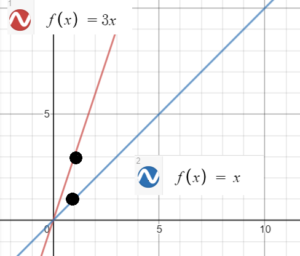# Linear Parent Function

The Linear Parent Function is f(x) = x. It is the most basic form of a linear function f(x) = mx + b.

## Linear Parent Function Characteristics

The Linear Parent Function has the following characteristics:

## Graph of the Linear Parent Function

The above graph shows the basic linear parent function f(x) = x, which creates a diagonal line when graphed. The function is the simplest linear function possible, with a = 1 and b = 0:

• f(x) = ax + b becomes f(x) = 1x + 0 or simply f(x) = x.

## Why is it called a “Parent Function?”

It’s called a “parent function” because every linear function in the Cartesian plane stems from the linear parent function f(x) = x. Every other possible linear function of the form y = mx + b is a child function of this parent. Together, the parent linear function and child functions make up a family: the family of linear functions.

To put this another way, every linear function f(x) = mx + b is a transformation of the linear parent function f(x) = x. For example, the function f(x) = 3x is the parent function vertically stretched by a factor of 3; Instead of the function passing through (1, 1) the graph passes through (3, 1):References

Beezer, Robert A. Linear Transformations. from A First Course in Linear Algebra, version 3.50. Created on December 12, 2015. Retrieved from http://linear.ups.edu/html/section-LT.html on November 9, 2019
Larson, R. & Edwards, B. (2009). Calculus. Cengage Learning.
Oldham, K. et al. (2008). An Atlas of Functions: with Equator, the Atlas Function Calculator 2nd Edition. Springer.

CITE THIS AS:
Stephanie Glen. "Linear Parent Function" From StatisticsHowTo.com: Elementary Statistics for the rest of us! https://www.statisticshowto.com/linear-parent-function/